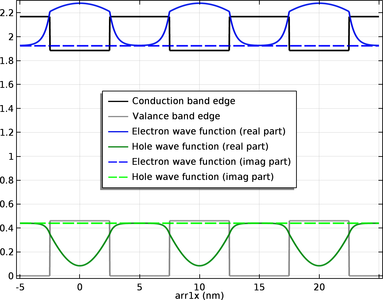# Application Gallery

## Superlattice Band Gap Tool

Application ID: 45281

The Superlattice Band Gap Tool model helps the design of periodic structures made of two alternating semiconductor materials (superlattices). The model uses the effective mass Schrödinger equation to estimate the electron and hole ground state energy levels in a given superlattice structure. Device engineers can use the model to quickly compute the effective band gap for a given periodic structure and iterate the design parameters until they reach a desired band gap value.

You can find out more about the model in this blog post: "Computing the Band Gap in Superlattices with the Schrödinger Equation"This model example illustrates applications of this type that would nominally be built using the following products: